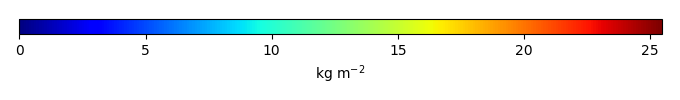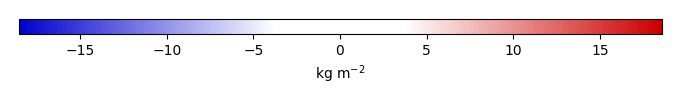# Mean State

Period Mean (original grids) [Pg]
Model Period Mean (intersection) [Pg]
Model Period Mean (complement) [Pg]
Benchmark Period Mean (intersection) [Pg]
Benchmark Period Mean (complement) [Pg]
Bias [kg m-2]
Bias Score 
Spatial Distribution Score 
Overall Score 
Benchmark [-] 30.1
CLM5PHSOFF [-] 543. 14.0 529. 29.9 0.195 -1.72 0.462 0.625 0.544
CLM5PHSON [-] 487. 22.3 465. 29.9 0.195 -0.481 0.575 0.774 0.674
Period Mean (original grids) [Pg]
Model Period Mean (intersection) [Pg]
Model Period Mean (complement) [Pg]
Benchmark Period Mean (intersection) [Pg]
Benchmark Period Mean (complement) [Pg]
Bias [kg m-2]
Bias Score 
Spatial Distribution Score 
Overall Score 
Benchmark [-] 1.44
CLM5PHSOFF [-] 11.6 4.77 6.63 1.41 0.0279 2.80 0.342 0.420 0.381
CLM5PHSON [-] 14.5 5.95 8.42 1.41 0.0279 4.18 0.208 0.467 0.337
Period Mean (original grids) [Pg]
Model Period Mean (intersection) [Pg]
Model Period Mean (complement) [Pg]
Benchmark Period Mean (intersection) [Pg]
Benchmark Period Mean (complement) [Pg]
Bias [kg m-2]
Bias Score 
Spatial Distribution Score 
Overall Score 
Benchmark [-] 0.00649
CLM5PHSOFF [-] 16.3 0.0151 15.5 0.00649 0.0419 0.442 0.265 0.354
CLM5PHSON [-] 10.2 0.0250 9.91 0.00649 0.233 0.584 0.753 0.668
Period Mean (original grids) [Pg]
Model Period Mean (intersection) [Pg]
Model Period Mean (complement) [Pg]
Benchmark Period Mean (intersection) [Pg]
Benchmark Period Mean (complement) [Pg]
Bias [kg m-2]
Bias Score 
Spatial Distribution Score 
Overall Score 
Benchmark [-] 17.4
CLM5PHSOFF [-] 7.11 5.92 0.831 17.3 0.0180 -2.78 0.488 0.618 0.553
CLM5PHSON [-] 12.0 11.6 0.244 17.3 0.0180 -1.06 0.651 0.854 0.753
Period Mean (original grids) [Pg]
Model Period Mean (intersection) [Pg]
Model Period Mean (complement) [Pg]
Benchmark Period Mean (intersection) [Pg]
Benchmark Period Mean (complement) [Pg]
Bias [kg m-2]
Bias Score 
Spatial Distribution Score 
Overall Score 
Benchmark [-] 1.52
CLM5PHSOFF [-] 32.6 1.92 30.7 1.46 0.0621 1.56 0.654 0.912 0.783
CLM5PHSON [-] 43.6 1.80 42.0 1.46 0.0621 3.72 0.646 0.903 0.774
Period Mean (original grids) [Pg]
Model Period Mean (intersection) [Pg]
Model Period Mean (complement) [Pg]
Benchmark Period Mean (intersection) [Pg]
Benchmark Period Mean (complement) [Pg]
Bias [kg m-2]
Bias Score 
Spatial Distribution Score 
Overall Score 
Benchmark [-] 9.78
CLM5PHSOFF [-] 1.45 1.30 0.251 9.71 0.0683 -2.34 0.403 0.207 0.305
CLM5PHSON [-] 3.04 2.93 0.149 9.71 0.0683 -1.73 0.481 0.415 0.448

# Temporally integrated period mean

BENCHMARK MEANMODEL MEANBIASBIAS SCORESPATIAL TAYLOR DIAGRAMMODEL COLORS# Spatially integrated regional mean

MODEL COLORS# All Models

BenchmarkCLM5PHSOFFCLM5PHSON